# Engineering Mechanics - Center of Gravity and Centroid

### Exercise :: Center of Gravity and Centroid - General Questions

• Center of Gravity and Centroid - General Questions
6.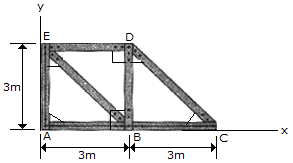The truss is made from seven members, each having a mass of 6 kg/m. Locate the position (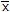,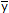) of the center of mass. Neglect the mass of the gusset plates at the joints.

 A.= 2.36 m,= 1.286 m B.= 2.33 m,= 1.333 m C.= 2.43 m,= 1.308 m D.= 2.79 m,= 1.757 m

Explanation:

No answer description available for this question. Let us discuss.

7.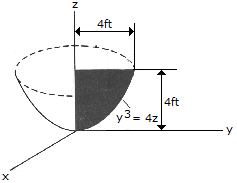Locate the center of gravity of the volume generated by revolving the shaded area about the z axis. The material is homogeneous.

 A.= 2.80 ft B.= 2.50 ft C.= 2.67 ft D.= 3.00 ft

Explanation:

No answer description available for this question. Let us discuss.

8.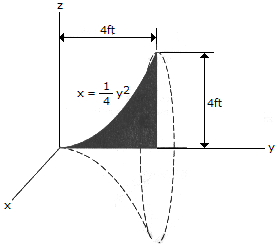Locate the center of gravity of the homogeneous "bell-shaped" volume formed by revolving the shaded area about the y axis.

 A.= 3.33 ft B.= 2.80 ft C.= 3.20 ft D.= 3.00 ft

Explanation:

No answer description available for this question. Let us discuss.

9.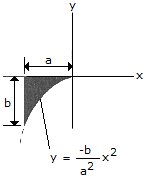Locate the centroid of the exparabolic segment of area.

 A.= -4a/5,= -b/4 B.= -3a/4,= -3b/10 C.= -2a/3,= -b/3 D.= -5a/7,= -3b/8